# Bihar Board 12th Physics Model Question Paper 3 in English Medium

Bihar Board 12th Physics Model Papers

## Bihar Board 12th Physics Model Question Paper 3 in English Medium

Instructions for the candidates :

1. Candidates are required to give answers in their own words as far as practicable.
2. Figures in the Right-hand margin indicates full marks.
3. While answering the questions, candidates should adhere to the words limit as far as practicable.
4. 15 Minutes of extra time has been allotted to read the questions carefully.
5. This question paper is divided into two sections : Section -A and Section-B
6. In Section A there are 35 Objective type questions which are compulsory, each carrying 1 mark. Darken the circle with Blue/Black ball pen against the correct option on OMR Sheet provided to you. Do not use Whitener/Liquid/ Blade/Nail on OMR Sheet, otherwise, your result will be treated as invalid.
7. In section-B, there are 18 Short answer type questions (each carrying 2 marks), out of which only 10 (ten) questions are to be answered Apart from this there are 06 Long Answer type questions each carrying 5 marks, out of which 3 questions are to be answered.
8. Use of any electronic device is prohibited.Time : 3 Hours 15 Minute
Total Marks : 70

Objective Type Questions

There are 1 to 35 objective type questions with 4 options. Choose the correct option which is to be answered on OMR Sheet. (35 x 1 = 35)

Question 1.
Torque acting on dipole in electric field E is
$$(a) \overrightarrow{\mathrm{p}} \times \overrightarrow{\mathrm{E}} (b) \overrightarrow{\mathrm{P}} \cdot \overrightarrow{\mathrm{E}} (c) \overrightarrow{\mathrm{E}} \times \overrightarrow{\mathrm{P}} (d) P/E$$
$$(a) \overrightarrow{\mathrm{p}} \times \overrightarrow{\mathrm{E}}$$

Question 2.
The algebraic sum of currents meeting at a point in the electric circuit becomes
(a) Infinity
(b) Positive
(c) Zero
(d) Negative
(c) ZeroQuestion 3.
The density of metal is d and sp. resistance is r. It is in the form of wire whose length is 1 and resistance is R. The resistance mass of metal should be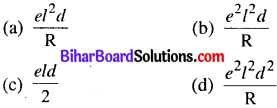(a)

Question 4.
The emf of cell is measured by
(a) Voltmeter
(b) Ammeter
(c) Galvanometer
(d) Potentiometer
(d) Potentiometer

Question 5.
The balancing point is obtained in potentiometer by increasing the length of wire
(a) On minimum length
(b) On maximum length
(c) On the same length
(d) Indefinite
(b) On maximum lengthQuestion 6.
If two cells of E1 and E2 are balanced at length l1 and l2 in potentiometer wire then(b)

Question 7.
The phase difference between current and emf in the condition of resonance is
(a) π/2
(b) π/4
(c) π
(d) zero
(d) zero

Question 8.
A copper ring lies in horizontal place. A bar magnet lies along its axis above the ring. The bar magnet is now released then
(a) Acceleration of the bar will be ‘g’
(b) Copper ring will go an cooling
(c) The acceleration of bar will be less than g
(d) The velocity of the bar will become upward
(a) Acceleration of the bar will be ‘g’

Question 9.
The induced emf of rod is
(a) BLV
(b) B2L2V
(c) Zero
(d) None of these
(c) ZeroQuestion 10.
The energy due to flow of current I in inductance L is
$$(a) Zero (b) \frac{1}{2} \mathrm{LI}^{2} (c) \frac{1}{2} \mathrm{LI} (d) \frac{1}{2} \mathrm{L}^{2} \mathrm{I}^{2}$$
(a) Zero
$$(b) \frac{1}{2} \mathrm{LI}^{2}$$

Question 11.
The power loss in A.C. circuit is
(a) only resistance
(b) only capacity
(c) only inductance
(d) All of these
(a) only resistance

Question 12.
The unit of mutualinductance is
(a) Weber
(b) Ohm
(c) Henry
(d) Gauss
(c) HenryQuestion 13.
If the real and apparent power of A.C. circuit is PT and PA respectively then power factor is
$$(a) \frac{P_{T}}{P_{A}} (b) \mathrm{P}_{\mathrm{T}} \times \mathrm{P}_{\mathrm{A}} (c) \frac{\mathrm{P}_{\mathrm{A}}}{\mathrm{P}_{\mathrm{T}}} (d) \mathrm{P}_{\mathrm{A}}+\mathrm{P}_{\mathrm{T}}$$
$$(a) \frac{P_{T}}{P_{A}}$$

Question 14.
Which have minimum wave length in the following?
(a) x-ray
(b) y-ray
(c) Micro wave
(b) y-ray

Question 15.
A Thin prism $$\left(\mu=\frac{3}{2}\right)$$ in air is immersed in water $$\left(\mu=\frac{6}{4}\right)$$. The ratio of angle of deviation in both condition will be
$$(a) \frac{15}{8} (b) \frac{8}{15} (c) \frac{5}{2} (d) \frac{2}{5}$$
$$(c) \frac{5}{2}$$

Question 16.
The refractive index of material of prism is ^3 • The minimum deviation for this prism is :
(a) 30°
(b) 37°
(c) 45°
(d) 60°
(d) 60°Question 17.
The cause of coloured thin film is
(a) Scattering
(b) Interference
(c) Dispersion
(d) Diffraction
(b) Interference

Question 18.
The radius of curvature of double convex lens is 20cm and μ = 1.5 then its power is
(a) 5D
(b) 10D
(c) 25D
(d) 20D
(a) 5D

Question 19.
The vertical section of cornea is not symmetrical about their axis. The defects of vision in eye is called
(a) Short sightedness
(b) Hypermetropia
(c) Presbyopia
(d) Astigmatism
(c) Presbyopia

Question 20.
The radius of curvature of plane mirror is
(a) Infinity
(b) Zero
(c) +5 cm
(d) -5 cm
(a) InfinityQuestion 21.
The ratio of intensity of two waves is 9:1 produces interference. The ratio of maximum and minimum intensity will be
(a) 10 : 8
(b) 9 : 1
(c) 4 : 1
(d) 2 : 1
(c) 4 : 1

Question 22.
If the frequency of photon incident on the metallic surface becomes double then maximum kinetic energy of emitted electrons will be
(a) double
(b) more than double
(c) not change
(d) none of these
(b) more than double

Question 23.
The photo electric effect supports
(a) Wave nature of light
(b) Particle nature of light
(c) Dual nature of light
(d) None of these
(a) Wave nature of light

Question 24.
The electron in hydrogen atom comes from third orbit to second orbit, then released energy is
(a) 1.5 leV
(b) 3.4 eV
(c) 1.89 eV
(d) 0.54 eV
(c) 1.89 eVQuestion 25.
The angular momentum of electron in hydrogen atom in lowest energy level is
$$(a) \frac{h}{\pi} (b) \frac{h}{2 \pi} (c) \frac{2 \pi}{h} (d) \frac{\pi}{h}$$
$$(b) \frac{h}{2 \pi}$$

Question 26.
In nuclear reactor the controlling rods are made up
(b) of Uranium
(c) of Graphite
(d) of Plutonium

Question 27.
Which is unstable?
(a) neutron
(b) proton
(c) electron
(d) α-particle
(a) neutronQuestion 28.
The Boolean expression of NOR gate is
(a) A + B = y
(b) $$\overline{\mathrm{A.B}}=y$$
(c) A.B = y
(d) $$\overline{\mathrm{A+B}}=y$$
$$(d) [latex]\overline{\mathrm{A+B}}=y$$

Question 29.
In figure logic gate is(a) OR
(b) NOR
(c) NAND
(d) AND
(d) AND

Question 30.
The majority current carrier in p-type semiconductor is
(a) electron
(b) hole
(c) photon
(d) proton
(b) holeQuestion 31.
The semi conductor behaves at absolute temperature
(a) fully conductor
(b) fully insulator
(c) super conductor
(d) semi conductor
(b) fully insulator

Question 32.
The conduction band are empty in
(a) Insulator
(b) Semi conductor
(c) In metal
(d) None of these
(b) Semi conductor

Question 33.
Which is not the example of digital communications?
(a) E-mail
(b) Cellular phone
(c) Television
(d) Communication satellite
(c) TelevisionQuestion 34.
The value of amplitude modulation index is
(a) Always zero
(b) between 1 and
(c) Between 0 and 1
(d) Always infinity
(c) Between 0 and 1

Question 35.
The relation between a and P in transistor is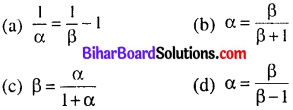(b)

Non-Objective Type Questions

Q.No. 1 to 18 are short answers type question. Each question carries 2 marks. Answer any ten (10) question.

Question 1.
What is Electrostatic shielding?
The electrostatic shielding is the best way to protect some area in vaccum from the influence of external electric field. ,
The technique of electrostatic shielding is applied basically in designing the cable for television.Question 2.
What is polar and non polar dielectrics?
Non-Polar dielectric :
A dielectric, in the atom or molecule of which, the centre of gravity of positive and negative charge coincide is called non-polar dielectric. Due to zero separation between +ve and -ve charges the electric dipole moment of such atom or molecules is zero.

Polar dielectric :
A dielectric, in the atom or molecules of which the centre of gravity of +ve and -ve charge do not coincide, is called polar dielectric

eg-NH3, HC1, H2O, CO2 etc.

Due to finite separation +ve and -ve charges, such atoms or molecules possess a finite electric dipole moment.

Question 3.
What is current density? Write its S.I. unit and dimension.
Current per unit area is called current density. It is denoted by ‘J’
$$\mathrm{J}=\frac{1}{\mathrm{A}}$$

It is a vector quantity
S.I. unit : Ampere/m2
Dimension : [M°L-2 T°]Question 4.
Write two factors on which resistivity of conductor depends.
The resistivity of conductor depends upon following factors :

• It depends upon temperature,
• It depends upon nature of substance of conductor.

Question 5.
Calculate the value of I in given circuit.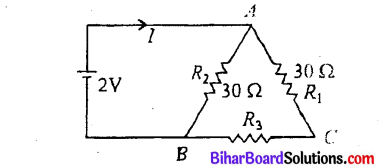According to figure
R1 and R2 are in series
Rs = R1 + R2
Rs = 30 + 30 = 60Ω
Now, R3 and Rs are in parallelQuestion 6.
Write Lenz’s law for electromagnetic induction.
Lenz’s law: This law states that whenever electric current is produced in circuit or in coil due to electromagnetic induction then it opposes that causes due to which it is produced.
$$\mathrm{e}=-\mathrm{n} \frac{\mathrm{d} \phi}{\mathrm{dt}}$$
e = induced emf

This law obeys the principle of Conservation of Energy.

Question 7.
Write two applications of ultraviolet waves.

• They are used to preserve food stuff and make drinking water free from bacteria, as these rays can kill bacteria, germs etc.
• They are used for sterlizing surgical instruments.Question 8.
The power of two lens are 12 D and -2D are kept in contact. What is the focal length of combination.?
Power of combination P = P1 + P2
P = 12 – 2 = 10
Focal length of combination
$$f=\frac{1}{p}=\frac{1}{10} \times 100=10 \mathrm{cm} ; f=10 \mathrm{cm}$$

Question 9.
Write Brewter’s law.
This law states that when the unpolarised light is incident on the surface on polarising angle then refractive index of medium is equal to tangent of polarising angle.
$$\mu=\tan i_{p}$$

Question 10.
State properties of photon.
Properties of photon are as follows :

• A photon travels with a speed of light in vacuum.
• A photon has zero rest mass.Question 11.
State two uses of photo diode.

• They are used as photo detectors to detect intensity of radiation.
• They are used as light operated switches.

Question 12.
What is Binding Energy per nucleon?

• The binding energy per nucleon of a nucleus is the binding energy divided by the total number of nucleons.

B.E./Nucleon =
$$\frac{\Delta m \times 931 \mathrm{ev}}{\Delta}$$

Question 13.
What are limitations of amplitude modulation?

• The quality of audio signal is not good,
• The atmospheric and industrial noise affect the audio signal,
• The efficiency of amplitude modulation is low.Question 14.
Write the differences between N-type and P- type semi-conductor.
N-type :

• when pentavalent impurity atom like As, Sb etc. are added in the intrinsic semi-conductor we get N- type of semi-conductor.
• The majority carriers in the N-type semi-conductor are electrons and minority carriers are hole.
• ne >> n0

P-type:

• When invalent impurity atoms like Gallium, Indium etc. are added in the intrinsic semi-condcutor we get p-type of semi conductors.
• The majority carriers in P-type semiconductor are holes and minority carriers are electrons.
• nh >>ne

Question 15.
What are base band and band width?
Base Band:
In telecommunication and signal processing it describes channel and system what range of frequency is measured from zero Hz to cut off frequency.Band Width :
It refers to the frequency range our which as equipotent rates on the portion of spectrum occupied by the signal.

Question 16.
Define Electric Intensity. Write its S.I. unit and dimension.
Electric Intensity :
The electric intensity at a point in the electric field is defined as the force which is experienced by unit positive charge placed at the point. It is denoted by $$\vec{E}$$
$$\vec{E}=\frac{\vec{F}}{q_{0}}$$

S.I. unit : Newton/Coulomb
or
Volt/metre
Dimension : [MLT3I-1]

Question 17.
Write properties of electric lines of force.

• The electric lines of force originated from positive charge and terminate on negative charge.
• The electric lines of force never intersect to each other.Question 18.
Why internal resistance of secondary cell is low?
As in secondry cell, distance between two plates is greater and their size are big. There is no polarisation in secondary cell. Due to this, internal resistance of secondary¬cell is 10w.

Q.No. 19 to 24 are long answers type question. Each question of this category carries 5 marks. Answer any three (3) question. (3 x 5 = 15)

Question 19.
State and Prove Gauss’s Theorem in electrostatics.
Statement of Gauss’s Theorem : The net- outward normal electric flux through any closed surface of any shape is equal to l/ε0 times the total charge contained within that surface i.e.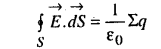where indicates the surface integral over the whole

of the closed surface. Σq is the algebraic sum of all the charges (i.e. net charge in Coulombs) enclosed by surface S.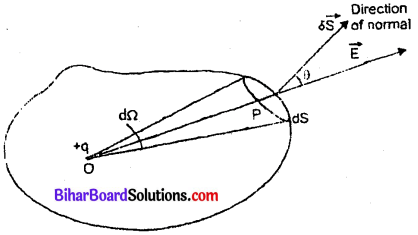Proof of Gauss’s Theorem :
Let a point charge + q coulomb be placed at O within the closed surface. Let E be the electric field strength at P. Let OP=r and the permitivity of free space or vacuum be e0.

Consider a small area’ $$\overrightarrow{d S}$$ of the surface surrounding the point P. Then the electric flux through $$\overrightarrow{d S}$$ is given bysubtended by area dS at point O. Here 0 is the angle between $$\overrightarrow{d S}$$ and $$\overrightarrow{E}$$Hence electric flux through whole of the closed surface.
$$\phi=\oint_{S} \overrightarrow{\mathrm{E}} \cdot \overrightarrow{\mathrm{dS}}=\frac{q}{4 \pi \varepsilon_{0}} \times \oint d \Omega$$

But $$\oint d \Omega$$ is the solid angle due to the entire closed surface S at an internal point O = 4π
∴ $$\quad \phi=\frac{q}{4 \pi \varepsilon_{0}} \cdot 4 \pi=\frac{1}{\varepsilon_{0}} q$$

It there are several charges, + q1, + q2, + q3 + q1 – q2 – q3 ………… inside the closed surface, each will contribute to the total electric flux. For positive charges the flux will be outward and hence positive; for negative charges the flux will be inward and negative. Therefore, the total electric flux in such a casewhere Zq is the algebraic sum of the charges within the closed surface.

Hence total electric flux through any closed surface is equal to l/e0 times the total charge (in Coulomb) enclosed within the surface which is Gauss’s law.Question 20.
Find an expression for the capacitance of a parallel plate capacitor when a dielectric slab of dielectric constant K and thickness $$t=\frac{d}{2}$$ but of same area on the plates is inserted between the capacitor plate. (d = separation between the plates).
Consider a paralled plate capacitor, area of each plate being A, the separation between the plates being d. Let a dielectric slab of dielectric constant K and thickness t < d be placed between the plates. The thickness of air between the plates is (d – t). If charges on plates are + Q and -Q, then surface charge densityThe electric field between the plates in air,
$$\mathrm{E}_{1}=\frac{\sigma}{\varepsilon_{0}}=\frac{Q}{\varepsilon_{0} \mathrm{A}}$$The electric field between the plates in slab,
$$\mathrm{E}_{2}=\frac{\sigma}{\mathrm{K} \varepsilon_{0}}=\frac{Q}{\mathrm{K} \varepsilon_{0} \mathrm{A}}$$

∴ The potential difference between the plates VAB = work done in carrying unit positive charge from one plate to another
= Σex (as field between the plates is not constant).∴ Capacitance of capacitor.Question 21.
State Biot-Savart law, giving the mathematical expression for it.
Use this law to derive the expression for the magnetic field due to a circular coil carrying current at a point along it axis.
How does a circular loop carrying current behave as a magnet?
(i) Magnetic field at the axis of a circular loop : Consider a circular loop of radius R carrying current I, with its plane perpendicular to the plane of paper. Let P be a point of observation on the axis of this circular loop at a distance x from its centre O. Consider a small element of length dl of the coil at point A. The magnitude of the magnetic induction $$\overrightarrow{\mathrm{dB}}$$ at point P due to this element is given byThe direction of $$\overrightarrow{\mathrm{dB}}$$ is perpendicular to the plane containing $$\overrightarrow{\mathrm{dl}}$$ and $$\overrightarrow{\mathrm{r}}$$ is given by right hand screw rule. As the angle between $$\overrightarrow{\mathrm{dl}}$$ and $$\overrightarrow{\mathrm{r}}$$ is 90°, the magnitude of the magnetic induction $$\overrightarrow{\mathrm{dB}}$$ is given by,
$$\overrightarrow{\theta B}=\frac{\mu_{0} t}{4 \pi} \frac{d \sin 9 \sigma^{0}}{r^{2}}=\frac{\mu_{0} d t^{2}}{4 \pi^{2}}$$ …….(ii)If we consider the magnetic induction produced by the whole of the circular coil, then by symmetry the components of magnetic induction perpendicular, to the axis will be cancelled out, while those parallel to the axis will be added up. Thus the resultant magnetic induction $$\overrightarrow{\mathrm{B}}$$ at axial point P is along the axis and may be evaluated as follows :

The component of $$\overrightarrow{\mathrm{dB}}$$ along the axis,Therefore the magnitude of resultant magnetic induction at axial point P due to the whole circular coil is given by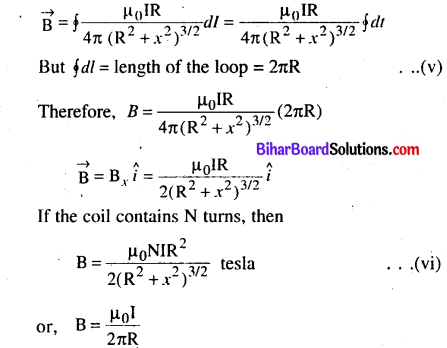Question 22.
Derive an expression for the force per unit length between two long straight parallel current carrying conductors. Hence define S.I. unit of current (ampere).
Suppose two long thin straight conductors (or wires) PQ and RS are placed parallel to each other in vacuum (or air) carrying currents I1 and I2 respectively. It has been observed experimentally that when the current in the wire are in the same direction, they experience an attractive force (fig. a) and when they carry currents in opposite directions, they experience a repulsive force (fig. b). Let the conductors PQ and RS carry currents I1 and I2 in same direction and placed at separation r. (fig). Consider a current-element ‘ab’ of length AL of wire RS. The magnetic field produced by current-carrying conductor PQ at the location of other wire RS.$$\mathrm{B}_{1}=\frac{\mu_{0} \mathrm{l}_{1}}{2 \pi r}$$ ……………………… (i)

According to Maxwell’s right hand rule or right hand palm rule no. I, the direction of B l will be perpendicular to the plane of paper and directed downward. Due to this magnetic field, each element of other wire experiences a force. The direction of current element is perpendicular to the magnetic field; therefore the magnetic force on element ab of length ΔL.
$$\Delta F=\mathrm{B}_{x} \mathrm{I}_{2} \Delta \mathrm{L} \sin 90^{\circ}=\frac{\mu_{0} \mathrm{I}_{1}}{2 \pi r} \mathrm{I}_{2} \Delta \mathrm{L}$$

∴ The total force on conductor of length L will be
$$\mathrm{F}=\frac{\mu_{0} \mathrm{I}_{1} \mathrm{I}_{2}}{2 \pi r} \Sigma \Delta \mathrm{L}=\frac{\mu_{0} \mathrm{I}_{1} \mathrm{I}_{2}}{2 \pi r} \mathrm{L}$$

∴ Force acting on per unit length of conductor
$$f=\frac{F}{L}=\frac{\mu_{0} I_{1} I_{2}}{2 \pi r} N / m$$

According to Flemming’s left hand rule, the direction of magnetic force will be towards PQ i.e., the force will be attractive.On the other hand if the currents I1 and I2 in wires are in opposite directions, the force will be repulsive. The magnitude of force in each case remains the same.Definition of S.I. unit of Current (ampere):
In S.I. system of fundamental unit of current ‘ampere’ has been define assuming the force between the two currents carrying wires as standard.

The force between two parallel currents carrying conductors of separation r is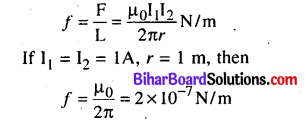Thus I ampere is the current which when flowing in each of parallel conductors placed at separation I m in vacuum exert a force of 2 x 10-7 on 1 m length of either wire.

Question 23.
With the help of a ray diagram, show the formation of image of a point object by refraction of light at a spherical surface separating two media of refractive indices n1 and n2 (n2 > n1), respectively. Using this diagram, derive the relation $$\frac{n_{2}}{v}-\frac{n_{1}}{u}=\frac{n_{1}-n_{1}}{R}$$

Write the sign conventions used. What happens to the focal length of convex lens when it is immersed in water?
Formula for Refraction at Spherical Surface Concave Spherical Surface :
Let SPS’ be a spherical refracting surface, which separates media ‘1’ and ‘2’. Medium ‘ 1’ is rarer and medium ‘2’ is denser. The refractive indices of media ‘1’ and ‘2’ are n1 and n2 respectively (n1 < n2). Let P be the pole and C the centre of curvature and PC the principal axis of spherical refracting surface.O is a point-object on the principal axis. An incident ray OA, after refraction at A on the spherical surface bends towards the normal OAN and moves along AB. Another incident ray OP falls on the surface normally and hence passes undeviated after refraction. These two rays, when produced backward meet at point I on principal axis. Thus I is the virtual image of O.Let angle of incidence of ray OA be / and angle of refraction be r i.e.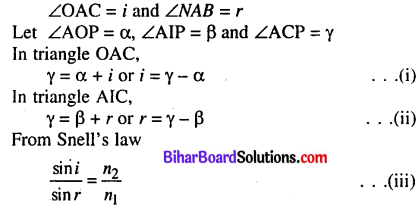If point A is very near to P, then angles i, r, a, P, Y will be very small, therefore sin i = i and sin r = r
From equation (iii)
$$\frac{i}{r}=\frac{n_{2}}{n_{1}}$$
Substituting values of i and r from (i) and (ii) we get
$$\frac{\gamma-\alpha}{\gamma-\beta}=\frac{n_{2}}{n_{1}} \text { or } n_{1}(\gamma-\alpha)=n_{2}(\gamma-\beta) \quad \ldots \text { (iv) }$$

The length of perpendicular AM dropped from A on the principal axis is h, i.e. AM = h. As angles a, P and y are very small, therefore
tana = α, tan β = β, tan γ = γ
Substituting these values in equation (iv)
n1 (tanY-tanoO = /j2(tanY-tanP) ……………. (v)
As point A is very close to P, point M is coincident with FLet u, v and R be the distance of object O, image I and centre of curvature C from pole P. By sign convention PO, PI and PC are negative i.e. u = – PO, v = – PI and R = – PC

Substituting these values in (vii) we getQuestion 24.
Derive Einstein’s photoelectric equation ^
$$\frac{1}{2}$$
$$m v^{2}=h v-h v_{0}$$
Einstein’s Explanation of Photoelectric Effect: Einstein’s Photoelectric Equation :

Einstein explained photoelectric effect on the basis of quantum theory.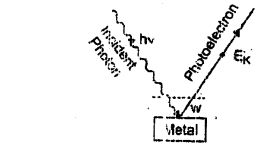The main points are :

1. Light is propagated in the form of bundles of energy. Each bundle of erergy is called a quantum or photon and has energy hv, where h = Plank’s constant and v = frequency of light.2. The photoelectric effect is due to collision of a photon of incident metal light and a bound electron of the metallic cathode.

3. When a photon of incident light falls on the metallic surface, it is completely absorbed. Before being absorbed it penetrates through a distance of nearly 10-8 m (or 100 A). The absorbed photon transfers its whole energy to a single electron. The energy of photon goes in two parts : a part of energy is used in releasing the electron from the metal surface (i.e., in overcoming work function) and the remaining part appears in the form of kinetic energy of the same electron.

If v be the frequency of incident light, the energy of photon = hv. If W be the work function of metal and Ek the maximum kinetic energy of photoelectron, then according to Einstein’s explanation.

hv = W + EK
or, EK = hv – W …………………….(i)

This is called Einstein’s photoelectric equation.

If v0 be the threshold frequency, then if frequency of incident light is less then v0, no electron will be emitted and if the frequency of incident light be v0, then Ek = 0; so from equation (i)
0 = hv0 – W or W = hv0

If λ0 be the threshold wavelength, then $$v_{0}=\frac{c}{\lambda_{0}}$$, where c is the speed of light in vacuum.

∴ Work function
$$\mathrm{W}=h v_{0}=\frac{h c}{\lambda_{0}}$$ ………………… (ii) Substituting this value in equation (i), we get
Ek = hv – hv0 ……………….(iii)
This is another form of Einstein’s photoelectric equation.